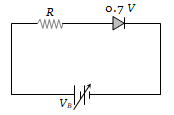# NEET Physics Semiconductor Electronics Questions Solved

The junction diode in the following circuit requires a minimum current of 1 mA to be above the knee point (0.7 V) of its I-V characteristic curve. The voltage across the diode is independent of current above the knee point. If ${\mathrm{V}}_{\mathrm{B}}$ = 5 V, then the maximum value of R so that the voltage is above the knee point, will be(a) 4.3 k$\mathrm{\Omega }$                 (b) 860 k$\mathrm{\Omega }$

(c) 4.3 $\mathrm{\Omega }$                   (d) 860 $\mathrm{\Omega }$